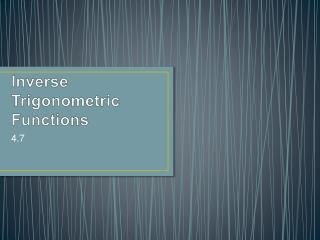DownloadDownload PresentationInverse Trigonometric Functions

# Inverse Trigonometric Functions

Télécharger la présentation## Inverse Trigonometric Functions

- - - - - - - - - - - - - - - - - - - - - - - - - - - E N D - - - - - - - - - - - - - - - - - - - - - - - - - - -
##### Presentation Transcript

1. Definition of Inverse Sine Function The inverse sine function is defined by y = arcsin x if and only if sin y = x where -1 ≤ x ≤ 1 and –π/2 ≤ y ≤ π/2. The domain of y = arcsin x is [-1, 1] and the range is [ -π/2 , π/2].

2. What does this mean? Remember from section 1.6 that for a function to have an inverse function, it must be one-to-one (pass the Horizontal Line Test). If you graph y = sin x if does not pass the Horizontal Line Test. But if you take a portion of the graph, say between –π/2 and π/2, it does pass the test. Also, • On the interval [-π/2, π/2] the function y = sin x is increasing. • On the interval [-π/2, π/2] y = sin x take on its full range of values -1 ≤ sin x ≤ 1. • On the interval [ -π/2, π/2] y = sin x is one-to-one. Sine y = sin x is one-to-one it has a unique inverse function called the inverse sine function denoted as y = arcsin x or y = sin-1 x. Arcsin x means the angle (or arc) whose sine is x.

3. Example 1: Evaluating the Inverse Sine Function If possible, find the exact value • Arcsin (-1/2) • Sin-1 • Sin-1 2

4. Example 2: Graphing the Arcsin Function Sketch a graph of y = arcsin x by hand.

5. Definition of the Inverse Trigonometric Functions FunctionDomainRange y = arcsin x -1 ≤ x ≤ 1 -π/2 ≤ y ≤π/2 y = arccos x -1 ≤ x ≤ 1 0 ≤ y ≤ π y = arctan x -∞ ≤ x ≤ ∞ -π/2 ≤ y ≤π/2 The graphs of these functions can be found on page 311.

6. Example 3: Evaluating Inverse Trig. Functions Find the exact values. • arc cos • Cos-1 (-1) • Arctan 0 • Tan-1 (-1)

7. Example 4: Calculators & Inverse Trigonometric Functions Use a calculator to approximate the value (if possible). • Arctan (-8.45) • Sin-1 (.2447) • Arccos 2

8. Compositions of Functions Remember from 1.6 that if a function has an inverse the following properties must be true. f(f-1(x)) = x f-1(f(x)) = x

9. Inverse Properties If -1 ≤ x ≤ 1 and –π/2 ≤ y ≤π/2 then sin(arcsin x) = x and arcsin(sin y) = y. If -1 ≤ x ≤ 1 and 0 ≤ y ≤ π then cos(arccos x) = x and arccos(cos y) = y. If x is a real number and –π/2≤ y ≤π/2 then tan(arctan x) = x and arctan(tan y) = y.

10. Example 5: Using Inverse Properties If possible, find the exact value. • tan[arctan(-5)] • Arcsin(sin(5π/3)) • Cos(cos-1π)